Select Page

# Introduction to Quantum Mechanics

## Dr Mark S. D. Read

### 1 Introduction

A wave function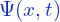is a function that satisfies a wave equation and describes the properties of a wave  . For a sound wave, the wave function is associated with the pressure at a timeand position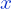. For a water wave,is the height of the wave at a time.

Quantum mechanics treats moving matter as a wave, called a ‘matter wave’ (by combining the classical wave equation and the de Broglie relation ). However, unlike the classical mechanics of wave motion, in quantum mechanics the wave amplitudeitself has no physical meaning. it is a complex quantity representing the variation of a matter wave.

#### 1.1 Born’s Statistical Interpretation

In 1926 Max Born suggested an interpretation of the ‘wavy nature’ of quantum particles as ‘waves of probability’. According to Born, the wave equation presented by Schrödinger the previous year was fundamentally a piece of mathematical machinery for calculating the chances of observing a particular outcome in an experiment. Essentially, Born’s rule connects quantum theory to experiment. “The Born rule is the crucial link between the abstract mathematical objects of quantum theory and the world of experience.”

The Born rule is a key postulate of quantum mechanics which gives the probability that measurement of a quantum system produces a given result. In its simplest form, it states that “the probability density of finding a particle at a given point is proportional to the square of the magnitude of the particle’s wave function at that point.”

Born’s postulate that the wave function, (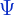) describing a particle’s behaviour is related to the probability of finding the particle may be represented mathematically by: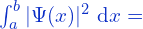{Probability of finding the particle betweenand} (1)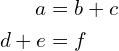Wave functions are commonly denoted by the variable.
 In 1924 Louis de Broglie suggested that a relationship that had been derived to relate momentum and wavelength for light should also apply to particles.{\color{red}

\section{Introduction}

A \textbf{wave function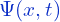} is a function that satisfies a wave equation and describes the properties of a wave\footnote{Wave functions are commonly denoted by the variable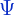}. For a sound wave, the wave function is associated with the pressure at a timeand position. For a water wave,is the height of the wave at a time.

Quantum mechanics treats moving matter as a wave, called a matter wave’ (by combining the classical wave equation and the de Broglie relation\footnote{In 1924 Louis de Broglie suggested that a relationship that had been derived to relate momentum and wavelength for light should also apply to particles.}). However, unlike the classical mechanics of wave motion, in quantum mechanics the wave amplitudeitself has no physical meaning. it is a complex quantity representing the variation of a matter wave.

\subsection{Born’s Statistical Interpretation}

In 1926 Max Born suggested an interpretation of the wavy nature’ of quantum particles as `waves of probability’. According to Born, the wave equation presented by Schr\”{o}dinger the previous year was fundamentally a piece of mathematical machinery for calculating the chances of observing a particular outcome in an experiment. Essentially, Born’s rule connects quantum theory to experiment. “The Born rule is the crucial link between the abstract mathematical objects of quantum theory and the world of experience.”

The Born rule is a key postulate of quantum mechanics which gives the probability that measurement of a quantum system produces a given result. In its simplest form, it states that “the probability density of finding a particle at a given point \textbf{is proportional to the square of the magnitude of the particle’s wave function} at that point.”

Born’s postulate that the wave function, () describing a particle’s behaviour is related to the probability of finding the particle may be represented mathematically by:

(1)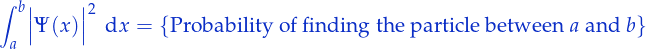The implication here is that the wave function itself does not represent the probability, but a probability amplitude, and that the information contained inonly represents the probability that one would measure a certain dynamical quantity, but cannot give pre-determined results in the same manner that deterministic classical mechanics will. Whereas classical mechanics is completely deterministic (if the initial conditions are known, the exact position where the particle will be at a later point in time can be predicted), quantum mechanics only provides statistical information about what the possible measurements will be. This interpretation, although since born out by much experimentation, caused much debate in the history of quantum mechanics.}